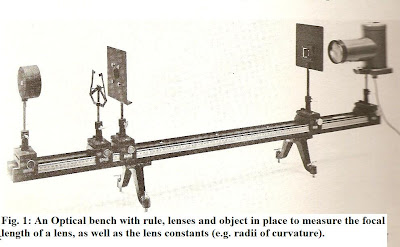## Thursday, June 16, 2011

### Introduction to Basic Physics (Thin Lenses) - Pt. 18We now turn to the investigation of thin lenses, a paramount practical investigation of refraction with applications in telescopes, mircoscopes, binocular, cameras and projectors as well as eye glasses.

Experimental set up: To Find Lens Constants:

This is shown in Fig. 1, for which an optical bench is used. The bench includes at least three sliding mounts, at least three lenses of differing focal lengths (e.g. 20 - 30 cm), an illuminated wire screen to serve as an object, a hooded screen, and am image screen with magnifier.

The theoretical results are depicted in the lower graphic, with the Object O positioned on the left side of the lens, which has a radius of curvature R2, and center of curvature C2, and an image I on the right side of the lens, which has a radius of curvature R1 and center of curvature C1. The object distance is then s, and the image distance is s'. These can then be used to form the thins lens formula (top) and the "lens makers equation" bottom.

Procedure:

The bench is set up as shown with 4 carrier slides or mounts, one for the object, one for the lens, one for the hooded screen and one for the light source. The lenses chosen for examination are all double convex (thus each will have R1, R2) and be made of ordinary crown glass.

Place the object screen near one end of the bench and the lens near the center, and take measurements: s abnd s'. Repeat then for a different value of s' such that s < s'. Take spherometer measurements of the lens, to find R1, and R2.

Calculations:

From the initial data s and s' the focal length f can be found (sing the thin lens equation). Using the values obtained for R1 and R2, the value for n, the refractive index, can be found.

Sample problem:

In the experimental set up shown in Fig. 1, a student obtains the following measurements:

R1 = 10 cm

R2 = -4 cm

s = 30 cm

a) If the student is given the refractive index of the lens as n = 1.52 how will he find the focal length?

b) Given the above (a) and the measurement made for s, how can the student find the image distance, s'?

Solution:

a) By the lens maker's eqn.

1/f = (n - 1) [1/R1 - 1/R2]

then: f = R1 R2/ (R2 - R1) x (1/n -1)

= [(10 cm) (- 4cm)]/ [-4 cm - 10 cm] x 1/0.520

f = (-40) cm/ -14 x 1/0.520 = 5.49 cm

b) Re-arrange the thin lens eqn. to make s' the subject:

1/s' = 1/f - 1/s or:

1/s' = s - f/ fs

then:

s' = fs / (s - f) = (5.49 cm)(30 cm)/ (30 cm - 5.49 cm)

s' = 6.72 cm

Other problems:

1) Using the experimental information and the lens diagram graphic as a guide, construct a lens diagram and thereby find the height of the image formed in the student's experiment.

2) By a similar graphical construction, find the position of the image formed by a converging lens (f = 15 cm) when:

a) the object is 60 cm from the lens

b) the object is 30 cm from the lens

3) A lamp and a screen are 160 cm apart. What should the focal length of the lens be in order to produce a real image of the lamp three times as large as the source?

(Hint: we can use the proportion: h'/h = s'/s to find image or object dimensions using image and object distances)